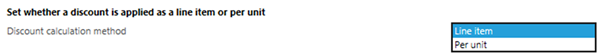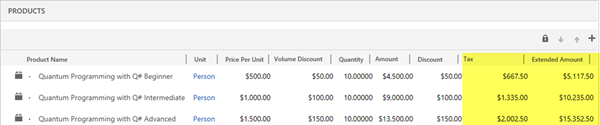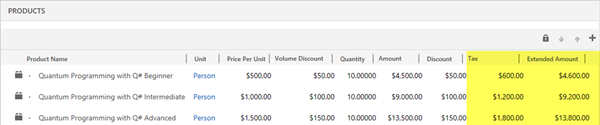# Discount Calculation Method for Product Line Items in Dynamics 365

### Colin Maitland, 15 November 2018

There are two methods that might be used by Microsoft Dynamics 365 for calculating the Extended Amount on Opportunity Products, Quote Products, Order Products and Invoice Products depending on the configuration of the Discount Calculation Method option in System Settings.

By default this option is set to ‘Line item’ but it can be changed to ‘Per unit’.The ‘Line item’ method does not multiply the Manual Discount Amount by the Quantity. The Per Unit method multiplies the Manual Discount Amount by the Quantity.

In the following examples, the Tax is 15% of the calculated non-tax amount.

The following image shows the calculation of the Extended Amount for Order Products using the ‘Line item’ Discount Calculation Method.The calculation of Extended Amount using the ‘Line item’ Discount Calculation Method is as follows:

•    (((Price Per Unit - Volume Discount) * Quantity) - Discount Amount) + Tax; e.g. ((\$1,000 - \$100) * 10) - \$100) + \$1,335 = \$10,235. In this example, the pre-Tax amount is \$8,900.

The following image shows the calculation of the Extended Amount for Order Products using the ‘Per unit’ Discount Calculation Method. The Tax is 15% of the calculated non-tax amount.The calculation of Extended Amount using the ‘Per unit’ Discount Calculation Method is as follows:

•    (((Price Per Unit - Volume Discount) * Quantity) - (Quantity * Discount Amount)) + Tax

e.g. ((\$1,000 - \$100) * 10) - (10 * \$100)) + \$1,200 = \$9,200. In this example, the pre-Tax amount is \$8,000.

If there was no tax on either of these two examples, the sum of the Extended Amount for the Order related Order Products would be \$26,700 when using ‘Line Item’ and \$24,000 when calculated using ‘Per Unit’.